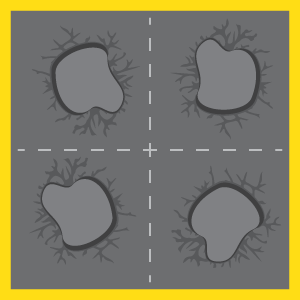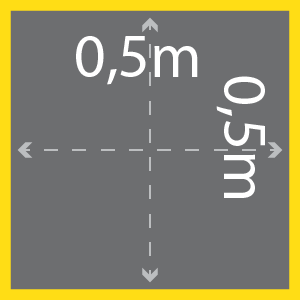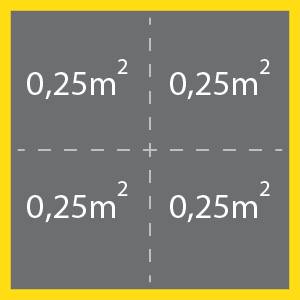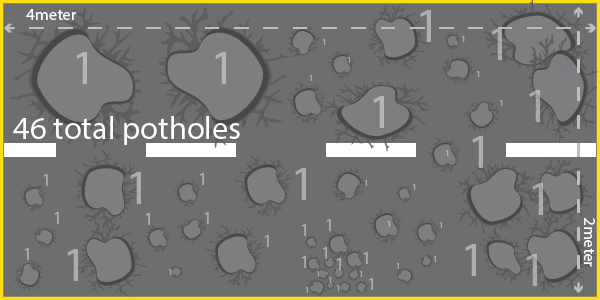# CALCULATE SQUARESThe Jetpatcher asphalt product  that gets blasted into a road failure, compacted from the bottom up, consists of aggregate and a special formulated bitumen emulsion. For the Jetpatcher technology, a small pothole and a big pothole is the same and gets fixed with the same tar mixture. The only difference when it comes to the size of the pothole is the application rate and quantity of the product.

Below follows a standardized formula to compare a big pothole to a small pothole so that their size matches and the correct amount of asphalt mixture is applied.  The global standard for measure is square meters and we wil convert it back to pothole counts.

## LENGTH x WIDTH = SQUARE M²### 1meter x 1meter = 1m²

Often more than usual we fix potholes smaller than 1 square meter and need to account for these road failures. Using the base of half a square by half a square provides a good mathematical equation to start counting potholes. Half a meter by Half a meter is not Half a square meter. It is a popular mistake to make.

#### 0.5meter x 0.5meter = 0.25m²This shows that within a 1m² patch we count 4 potholes

## 1m² ÷ 0.25m² = 4

In a 2m² patch we count 8 potholes.

Hence we measure the patch

## LENGTH x WIDTH = (SQUARE M²) x 4 = POTHOLE COUNT

The Illustrated piece of road have 46 visible road failures (potholes).## 4meter x 2 meter = 8m²## 8m² x 4 = 32 potholes